# SingleStore DB: Map Crimes, Visualize Hot Routes

## Abstract

Many great visualization techniques, such as kernel density mapping, can help us map and analyze crime concentrations. However, sometimes, it may be more beneficial to visualize crime concentrations along a linear network, such as a bus route or subway/underground line. Law enforcement agencies could use this to target resources at particular hot spots. To map these hot spots, we can use hot routes. Hot routes enable crimes to be mapped along sections of a linear network using thematic mapping (color and line width).

An excellent tutorial illustrates hot routes, using R, for the Bakerloo Line on the London Underground. Using Python, we’ll use the example described in that tutorial and apply it to SingleStore DB. In a previous article, we have already seen one approach to model and store the London Underground network in SingleStore DB.

The SQL scripts and notebook files used in this article are available on GitHub. The notebook files are available in DBC, HTML, and iPython formats.

## Introduction

This article will use SingleStore DB to help identify crime concentrations around the Bakerloo Line of the London Underground. We’ll use hot routes with thematic mapping. We’ll follow the following four-step process:

1. Prepare the network layer.
2. Link crime events to line segments.
3. Calculate a rate.
4. Visualize the results.

To begin with, we need to create a free Cloud account on the SingleStore website, and a free Community Edition (CE) account on the Databriks website. At the time of writing, the Cloud account from SingleStore comes with \$500 of Credits. This is more than adequate for the case study described in this article. For Databriks CE, we need to sign-up for the free account rather than the trial version. We are using Spark because, in a previous article, we noted that Spark was great for ETL with SingleStore DB.

We need to obtain three files. The data for the Bakerloo Line can be obtained from GitHub. The two files we need are:

• bakerloo_stops.csv: Contains Bakerloo Line station names and their latitude and longitude coordinates
• bakerloo_line.geojson: Contains a `LineString` with latitude and longitude coordinates for the entire Bakerloo Line

To match the original article with the implementation of the hot routes in R, we’ll download the same crime data for the British Transport Police (BTP). The file we need is 2020-02-btp-street.csv. This can be generated from the Data Downloads page. On that page, we’ll select the following:

• date range: February 2020 to February 2020.
• Forces: Check (✔) British Transport Police.
• Data sets: Check (✔) Include crime data.
• Generate file.

The download will be a zip file, and the CSV file we need can be extracted from that.

## Configure Databriks CE

A previous article provides detailed instructions on configuring Databriks CE with SingleStore DB. We can use those exact instructions for this use case. As shown in Figure 1, in addition to the SingleStore Spark Connector and the MariaDB Java Client jar file, we need to add GeoPandas and Folium. These can be added using PyPI.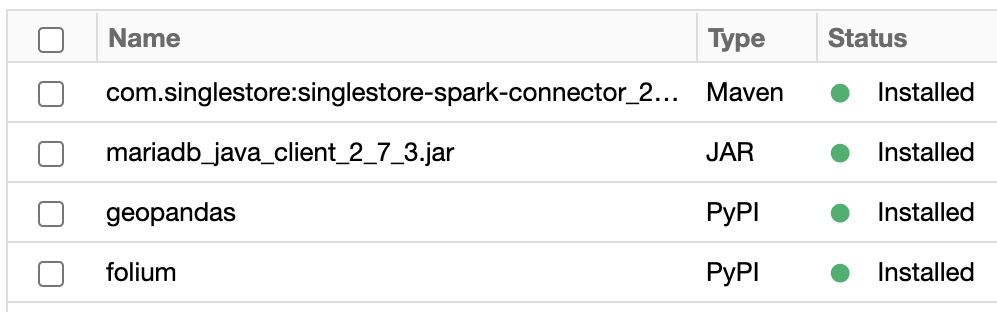Figure 1: Libraries

## Upload Data Files

To use the three data files, we need to upload them into the Databriks CE environment. A previous article provides detailed instructions on how to upload a data file. We can use those exact instructions for this use case.

## Create the Database Tables

In our SingleStore Cloud account, let’s use the SQL Editor to create a new database. Call this hot_routesas follows:

``CREATE DATABASE IF NOT EXISTS hot_routes;``

We’ll also create four tables, as follows:

``````USE hot_routes;

CREATE ROWSTORE TABLE bakerloo_stops (
stn_name VARCHAR(50),
geometry GEOGRAPHYPOINT
);

CREATE ROWSTORE TABLE bakerloo_sections (
geometry GEOGRAPHY
);

CREATE ROWSTORE TABLE crimes (
crime_type VARCHAR(100),
geometry GEOGRAPHYPOINT
);

CREATE ROWSTORE TABLE bakerloo_line_buff (
geometry GEOGRAPHY
);``````

SingleStore DB can store three main Geospatial types: Polygons, Paths and Points. In the above tables, GEOGRAPHY can hold Polygon and Path data. GEOGRAPHYPOINT can hold Point data.

1. The `bakerloo_stops` table contains the name of each station and its latitude and longitude.
2. The `bakerloo_sections` table contains two pairs of latitude and longitude coordinates for each section of the Bakerloo Line between two stations.
3. The `crimes` table contains details of crimes and their geospatial locations.
4. The `bakerloo_line_buff` table stores a Polygon that represents a buffer around the Bakerloo Line. This will become clear when we visualize it shortly.

## Data Loader for Hot Routes

Let’s now create a new Databriks CE Python notebook. We’ll call it Data Loader for Hot Routes. We’ll attach our new notebook to our Spark cluster.

First, let’s copy the three data files from FileStore to a temporary directory, as follows:

``````dbutils.fs.cp("/FileStore/bakerloo_stops.csv",
"file:///tmp/bakerloo_stops.csv")

dbutils.fs.cp("/FileStore/bakerloo_line.geojson",
"file:///tmp/bakerloo_line.geojson")

dbutils.fs.cp("/FileStore/2020_02_btp_street.csv",
"file:///tmp/2020_02_btp_street.csv")``````

### bakerloo_stops

Next, let’s read the `bakerloo_stops` file into a Pandas Dataframe and then choose the columns that we wish to keep, as well as describe how to create the geometry:

``````import pandas as pd
import geopandas as gpd

bakerloo_stops = gpd.GeoDataFrame(
df["stn_name"], geometry = gpd.points_from_xy(df.stn_lon, df.stn_lat), crs = "EPSG:4326"
)``````

Now, we’ll convert the geometry to a string format for our Spark Dataframe:

``````from shapely import wkt

bakerloo_stops["geometry"] = bakerloo_stops["geometry"].apply(wkt.dumps)``````

Next, we’ll create our Spark Dataframe:

``bakerloo_stops_df = spark.createDataFrame(bakerloo_stops)``

Now let’s check the Spark Dataframe:

``bakerloo_stops_df.show(5, False)``

The result should be similar to this:

``````+-------------+-----------------------------------------------+
|stn_name     |geometry                                       |
+-------------+-----------------------------------------------+
|Baker Street |POINT (-0.1571300000000000 51.5228830000000002)|
|Embankment   |POINT (-0.1226660000000000 51.5070580000000007)|
|Edgware Road |POINT (-0.1701500000000000 51.5202990000000014)|
|Kilburn Park |POINT (-0.1942320000000000 51.5349789999999999)|
|Lambeth North|POINT (-0.1123150000000000 51.4988079999999968)|
+-------------+-----------------------------------------------+
only showing top 5 rows``````

### bakerloo_line

Next, let’s read the `bakerloo_line` file:

``````bakerloo_line = gpd.GeoDataFrame.from_file("file:///tmp/bakerloo_line.geojson")

bakerloo_line.to_crs(epsg = 4326, inplace = True)``````

`bakerloo_line` is one long `LineString`, and we need to break it into segments. We can achieve this by applying a solution proposed on Stackoverflow, as follows:

``````from shapely.geometry import LineString

def segments(curve):
return list(map(LineString, zip(curve.coords[:-1], curve.coords[1:])))

bakerloo_line_segments = segments(bakerloo_line.geometry)``````

Now we can create a Dataframe:

``````bakerloo_sections = gpd.GeoDataFrame(
geometry = bakerloo_line_segments, crs = "EPSG:4326"
)``````

Convert the geometry to a string format for our Spark Dataframe:

``bakerloo_sections["geometry"] = bakerloo_sections["geometry"].apply(wkt.dumps)``

Next, we’ll create our Spark Dataframe:

``bakerloo_sections_df = spark.createDataFrame(bakerloo_sections)``

Now let’s check the Spark Dataframe:

``bakerloo_sections_df.show(5)``

The result should be similar to this:

``````+--------------------+
|            geometry|
+--------------------+
|LINESTRING (-0.33...|
|LINESTRING (-0.31...|
|LINESTRING (-0.30...|
|LINESTRING (-0.30...|
|LINESTRING (-0.29...|
+--------------------+
only showing top 5 rows``````

### crimes

Next, let’s read the `2020_02_btp_street` file into a Pandas Dataframe and then choose the columns we wish to keep, as well as describe how to create the geometry:

``````df = pd.read_csv("file:///tmp/2020_02_btp_street.csv")

crimes = gpd.GeoDataFrame(
df["Crime type"], geometry = gpd.points_from_xy(df.Longitude, df.Latitude), crs = "EPSG:4326"
)

crimes.rename(columns = {"Crime type" : "crime_type"}, inplace = True)``````

Now, we’ll convert the geometry to a string format for our Spark Dataframe:

``crimes["geometry"] = crimes["geometry"].apply(wkt.dumps)``

Next, we’ll create our Spark Dataframe:

``crimes_df = spark.createDataFrame(crimes)``

Now let’s check the Spark Dataframe:

The result should be similar to this:

``````+----------------+-----------------------------------------------+
|crime_type      |geometry                                       |
+----------------+-----------------------------------------------+
|Bicycle theft   |POINT (-0.2716040000000000 50.8344000000000023)|
|Bicycle theft   |POINT (-0.2716040000000000 50.8344000000000023)|
|Violence and ...|POINT (-0.2371240000000000 50.8325000000000031)|
|Public order    |POINT (-3.5594399999999999 54.6445000000000007)|
|Public order    |POINT (-1.3308100000000000 53.0182000000000002)|
+----------------+-----------------------------------------------+
only showing top 5 rows``````

### bakerloo_line_buff

We’ll now create a buffer around the `bakerloo_line` data:

``````bakerloo_line_buff = gpd.GeoDataFrame(
geometry = bakerloo_line.buffer(0.005), crs = "EPSG:4326"
)``````

Now, we’ll convert the geometry to a string format for our Spark Dataframe:

``bakerloo_line_buff["geometry"] = bakerloo_line_buff["geometry"].apply(wkt.dumps)``

Next, we’ll create our Spark Dataframe:

``bakerloo_line_buff_df = spark.createDataFrame(bakerloo_line_buff)``

Now let’s check the Spark Dataframe:

``bakerloo_line_buff_df.show()``

The result should be similar to this:

``````+--------------------+
|            geometry|
+--------------------+
|POLYGON ((-0.3144...|
+--------------------+``````

We are now ready to write the four Spark Dataframes to SingleStore DB. In the next code cell, we can add the following:

In the Setup notebook, we need to ensure that the server address and password have been added for our SingleStore DB Cloud cluster.

In the next code cell, we’ll set some parameters for the SingleStore Spark Connector, as follows:

``````spark.conf.set("spark.datasource.singlestore.ddlEndpoint", cluster)
spark.conf.set("spark.datasource.singlestore.disablePushdown", "false")``````

Finally, we are ready to write the Dataframes to SingleStore DB using the Spark Connector. First, `bakerloo_stops`:

``````(bakerloo_stops_df.write
.format("singlestore")
.mode("overwrite")
.save("hot_routes.bakerloo_stops"))``````

Then, `bakerloo_sections`:

``````(bakerloo_sections_df.write
.format("singlestore")
.mode("overwrite")
.save("hot_routes.bakerloo_sections"))``````

Next, `crimes`:

``````(crimes_df.write
.format("singlestore")
.mode("overwrite")
.save("hot_routes.crimes"))``````

Finally, `bakerloo_line_buff`:

``````(bakerloo_line_buff_df.write
.format("singlestore")
.mode("overwrite")
.save("hot_routes.bakerloo_line_buff"))``````

The overall quantity of data we are storing is relatively small. However, using SingleStore DB, we can scale our application to manage much larger networks, such as the entire London Underground System or the complete Overground Railway System in the United Kingdom.

Now that we have stored our data let’s begin creating a hot routes visualization.

## Hot Routes Visualization

Earlier in this article, we mentioned a four-step process, and to follow that process, let’s now create a new Databriks CE Python notebook. We’ll call it Hot Routes. We’ll attach our new notebook to our Spark cluster.

### 1. Prepare the Network Layer

In the first code cell, let’s add and execute the following:

In the following code cell, we’ll set some parameters for the SingleStore Spark Connector, as follows:

``````spark.conf.set("spark.datasource.singlestore.ddlEndpoint", cluster)
spark.conf.set("spark.datasource.singlestore.disablePushdown", "false")``````

Now we’ll load data from the `bakerloo_stops` table and store it in a GeoPandas Dataframe:

``````import geopandas as gpd
from shapely import wkt

.format("singlestore")
)

bakerloo_stops = df1.toPandas()
bakerloo_stops = gpd.GeoDataFrame(bakerloo_stops)
bakerloo_stops.set_crs(epsg = 4326, inplace = True)``````

We can create a quick visualization, as follows:

``````stops = bakerloo_stops.plot(color = "black", markersize = 15)

stops.set_axis_off()

stops.plot()``````

The result should be as shown in Figure 2.Figure 2: `bakerloo_stops`

Now we’ll load data from the `bakerloo_sections` table and store it in a GeoPandas Dataframe:

``````df2 = (spark.read
.format("singlestore")
)

bakerloo_sections = df2.toPandas()
bakerloo_sections = gpd.GeoDataFrame(bakerloo_sections)
bakerloo_sections.set_crs(epsg = 4326, inplace = True)``````

We can create a quick visualization, as follows:

``````sections = bakerloo_sections.plot(color = "#B36305", linewidth = 3)

sections.set_axis_off()

sections.plot()``````

The result should be as shown in Figure 3.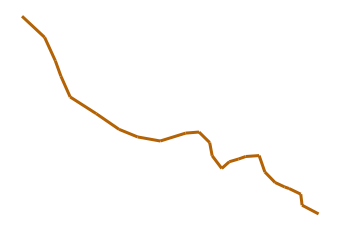Figure 3: `bakerloo_sections`

We can also combine both sets of data to produce a map of the Bakerloo Line:

``````base = bakerloo_sections.plot(color = "#B36305", linewidth = 3)

bakerloo_stops.plot(ax = base, color = "black", markersize = 15)

base.set_axis_off()

base.plot()``````

The result should be as shown in Figure 4.

: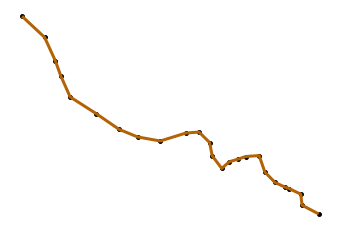Figure 4: Bakerloo Line Map

### 2. Link Crime Events to Line Segments

Now we’ll load data from the `crimes` table and store it in a GeoPandas Dataframe:

``````df3 = (spark.read
.format("singlestore")
)

crimes = df3.toPandas()
crimes = gpd.GeoDataFrame(crimes)
crimes.set_crs(epsg = 4326, inplace = True)``````

We can create a quick visualization as follows:

``````btp_crimes = crimes.plot()

btp_crimes.set_axis_off()

btp_crimes.plot()``````

The result should be as shown in Figure 5.Figure 5: UK Crime Data

Let’s now load data from the `bakerloo_line_buff` table and store it in a GeoPandas Dataframe:

``````df4 = (spark.read
.format("singlestore")
)

bakerloo_line_buff = df4.toPandas()
bakerloo_line_buff = gpd.GeoDataFrame(bakerloo_line_buff)
bakerloo_line_buff.set_crs(epsg = 4326, inplace = True)``````

We can create a quick visualization as follows:

``````base = bakerloo_line_buff.plot(
color = "lightgrey",
edgecolor = "black",
figsize = (10, 10)
)

base.set_axis_off()

base.plot()``````

The result should be as shown in Figure 6.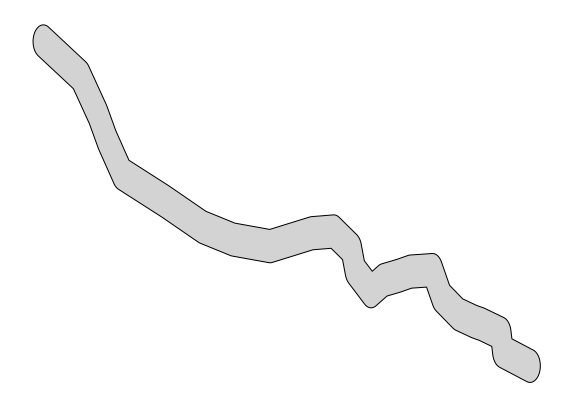Figure 6: `bakerloo_line_buff`

Now let’s narrow the number of `crimes` to those that lie within `bakerloo_line_buff`using a spatial intersection:

``````crimes = bakerloo_line_buff.overlay(
crimes, how = "intersection", keep_geom_type = False
)``````

We can also combine the four datasets to produce a visualization:

``````base = bakerloo_line_buff.plot(
color = "lightgrey",
edgecolor = "black",
figsize = (10, 10)
)

bakerloo_sections.plot(ax = base, color = "#B36305", linewidth = 3)

crimes.plot(ax = base, color = "red")

bakerloo_stops.plot(ax = base, color = "black", markersize = 15)

base.set_axis_off()

base.plot()``````

The result should be as shown in Figure 7.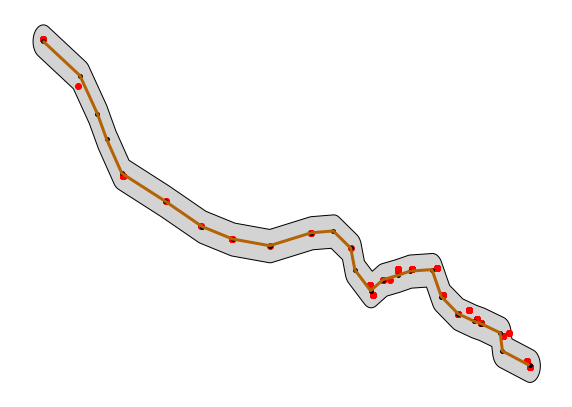Figure 7: Combined Datasets

Next, we need to find which section of the Bakerloo Line a particular crime is nearest. First, we’ll find the number of crimes:

``````bline_segments = gpd.sjoin_nearest(crimes, bakerloo_sections)

bline_segments.rename(
columns = {"index_right" : "segment"}, inplace = True
)``````

Then, we’ll sum them up for each section to provide the frequency:

``````sections_freq = gpd.GeoDataFrame(
bline_segments["segment"].value_counts()
)

sections_freq.rename(
columns = {"segment" : "freq"}, inplace = True
)``````

Next, we’ll get the geometry for each section by using a join and fill any sections that have no crimes with a 0 (zero):

``````bakerloo_sections = bakerloo_sections.join(sections_freq)

bakerloo_sections["freq"].fillna(0, inplace = True)``````

Now we can create a plot:

``````base = bakerloo_line_buff.plot(
color = "lightgrey",
edgecolor = "black",
figsize = (10, 10)
)

bakerloo_sections.plot(
ax = base,
column = "freq",
linewidth = 3,
cmap = "OrRd",
legend = True,
legend_kwds = {"label" : "Number of crimes", "orientation" : "horizontal"}
)

bakerloo_stops.plot(ax = base, color = "black", markersize = 15)

base.set_axis_off()

base.plot()``````

The result should be as shown in Figure 8.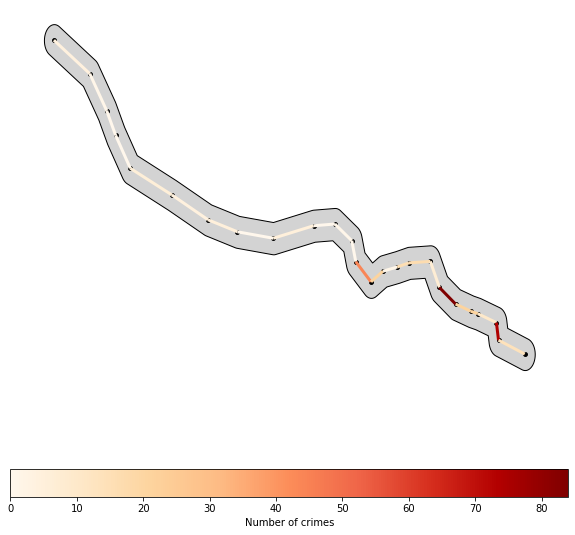Figure 8: Number of Crimes

### 3. Calculate a Rate

We need to consider the length of each section of the Bakerloo Line and then calculate the number of crimes per meter. First, we must switch the coordinate system to one that will enable us to determine the length of each section in metres:

``bakerloo_sections.to_crs(epsg = 3310, inplace = True)``

Then, we’ll create a new column to store the length:

``bakerloo_sections["length"] = bakerloo_sections["geometry"].length``

We’ll now switch back to the original coordinate system:

``bakerloo_sections.to_crs(epsg = 4326, inplace = True)``

We can now create a new column with the number of crimes per meter using the frequency and the length:

``bakerloo_sections["crime_per_m"] = bakerloo_sections["freq"] / bakerloo_sections["length"]``

### 4. Visualize the results

A plot will show the hot routes:

``````base = bakerloo_line_buff.plot(
color = "lightgrey",
edgecolor = "black",
figsize = (10, 10)
)

bakerloo_sections.plot(
ax = base,
column = "crime_per_m",
linewidth = 3 + bakerloo_sections["crime_per_m"] * 100,
cmap = "OrRd",
legend = True,
legend_kwds = {"label" : "Rate of crimes per metre", "orientation" : "horizontal"}
)

bakerloo_stops.plot(ax = base, color = "black", markersize = 15)

base.set_axis_off()

base.plot()``````

The result should be as shown in Figure 9.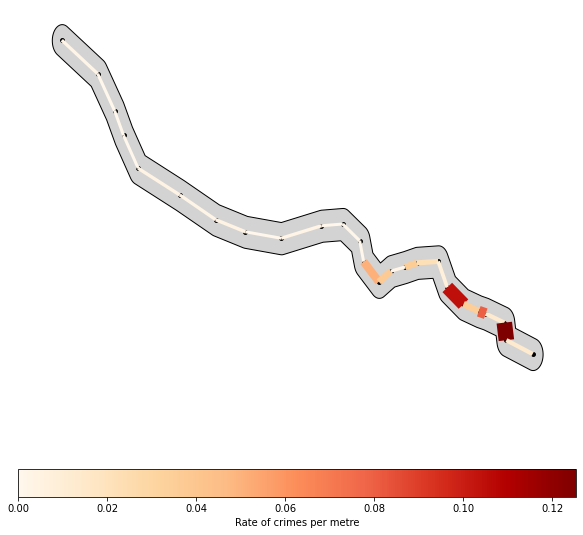Figure 9: Hot Routes

Bakerloo Line sections that are wider and darker in color contain more crimes per meter.

We can go a step further and use Folium to create a visualization:

``````import folium
from folium import plugins
from folium.plugins import HeatMap
from branca.colormap import linear

London = [51.509865, -0.118092]

m = folium.Map(location = London, tiles = "Stamen Terrain", control_scale = True, zoom_start = 14)

folium.GeoJson(
bakerloo_line_buff

HeatMap(
data = list(zip(crimes.geometry.y, crimes.geometry.x))

colormap = linear.OrRd_09.scale(
bakerloo_sections.crime_per_m.min(),
bakerloo_sections.crime_per_m.max()
)
colormap.caption = "Rate of crimes per metre"

for i in range(0, len(bakerloo_sections)):
x, y = bakerloo_sections.geometry.iloc[i].coords.xy
folium.PolyLine(
locations = [(y, x), (y, x)],
color = colormap(bakerloo_sections["crime_per_m"][i]),
weight = 3 + bakerloo_sections["crime_per_m"][i] * 100,
opacity = 1

stations_group = folium.FeatureGroup(name = "Stations").add_to(m)

for i in range(0, len(bakerloo_stops)):
icon = folium.Icon(
color = "red",
icon = "train",
icon_color = "white",
prefix = "fa"
),
location = [bakerloo_stops.geometry[i].y, bakerloo_stops.geometry[i].x],
popup = bakerloo_stops.stn_name[i],
))

crimes_group = folium.FeatureGroup(name = "Crimes").add_to(m)

for i in range(0, len(crimes)):
icon = folium.Icon(icon = "info-sign"),
location = [crimes.geometry[i].y, crimes.geometry[i].x],
popup = crimes["crime_type"][i]
))

plugins.Fullscreen(
position = "topright",
title = "Fullscreen",
title_cancel = "Exit",
force_separate_button = True

m``````

The result should be as shown in Figure 10.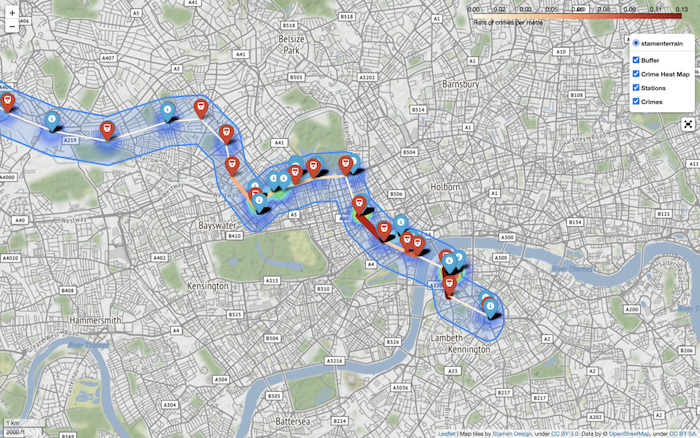Figure 10: Hot Routes using Folium

Using the menu in the top-right, we can select or deselect Buffer, Crime Heat Map, Stations and Crimes.

## Summary

This article has implemented hot routes for the Bakerloo Line using SingleStore DB and Python. SingleStore DB can also perform geospatial operations, as discussed in a previous article. Therefore, some of the operations that we needed to perform in Python could be replaced by SingleStore DB’s geospatial functions. In a future article, we’ll look to build another solution for hot routes that uses these product capabilities.

.